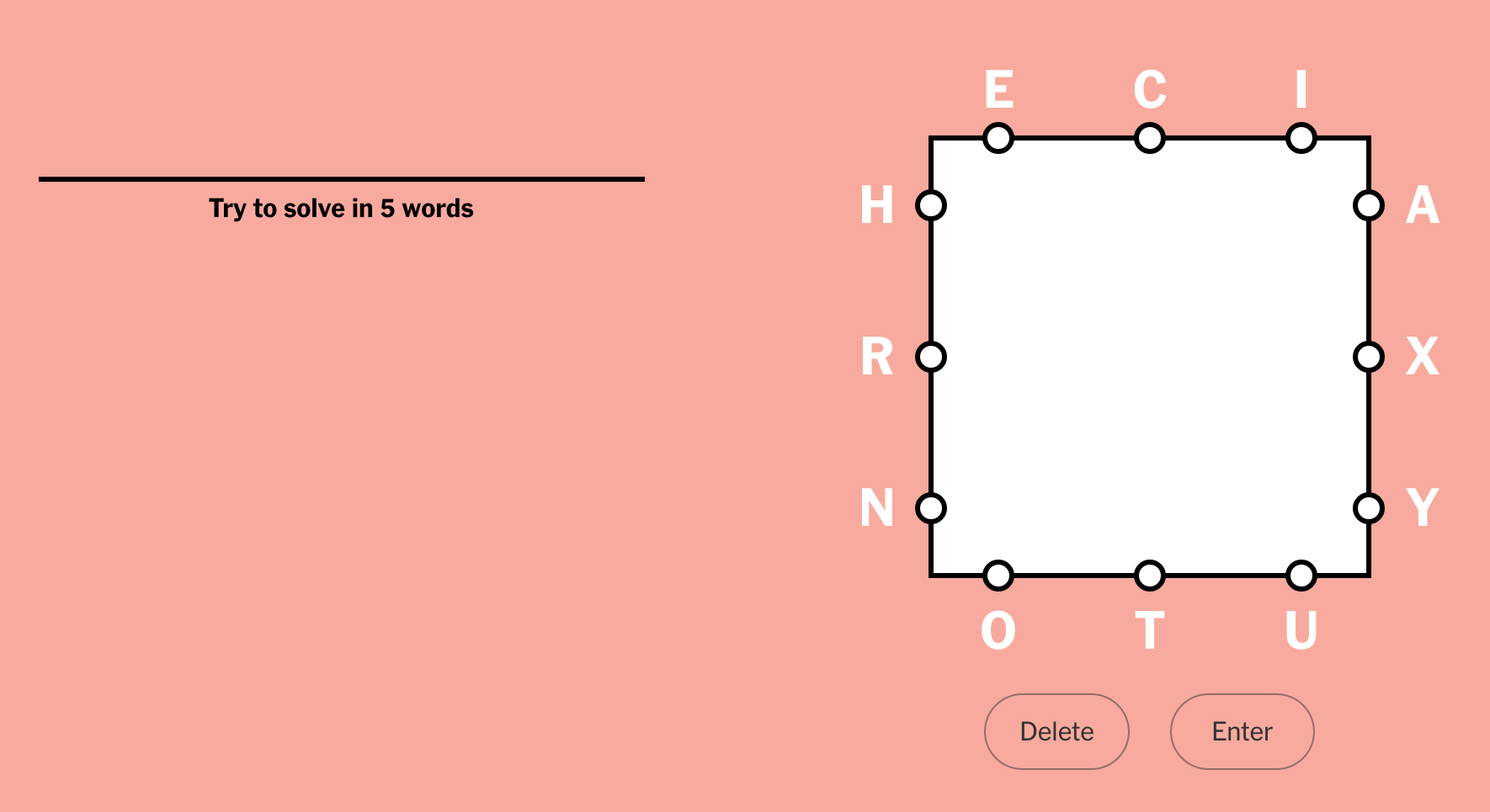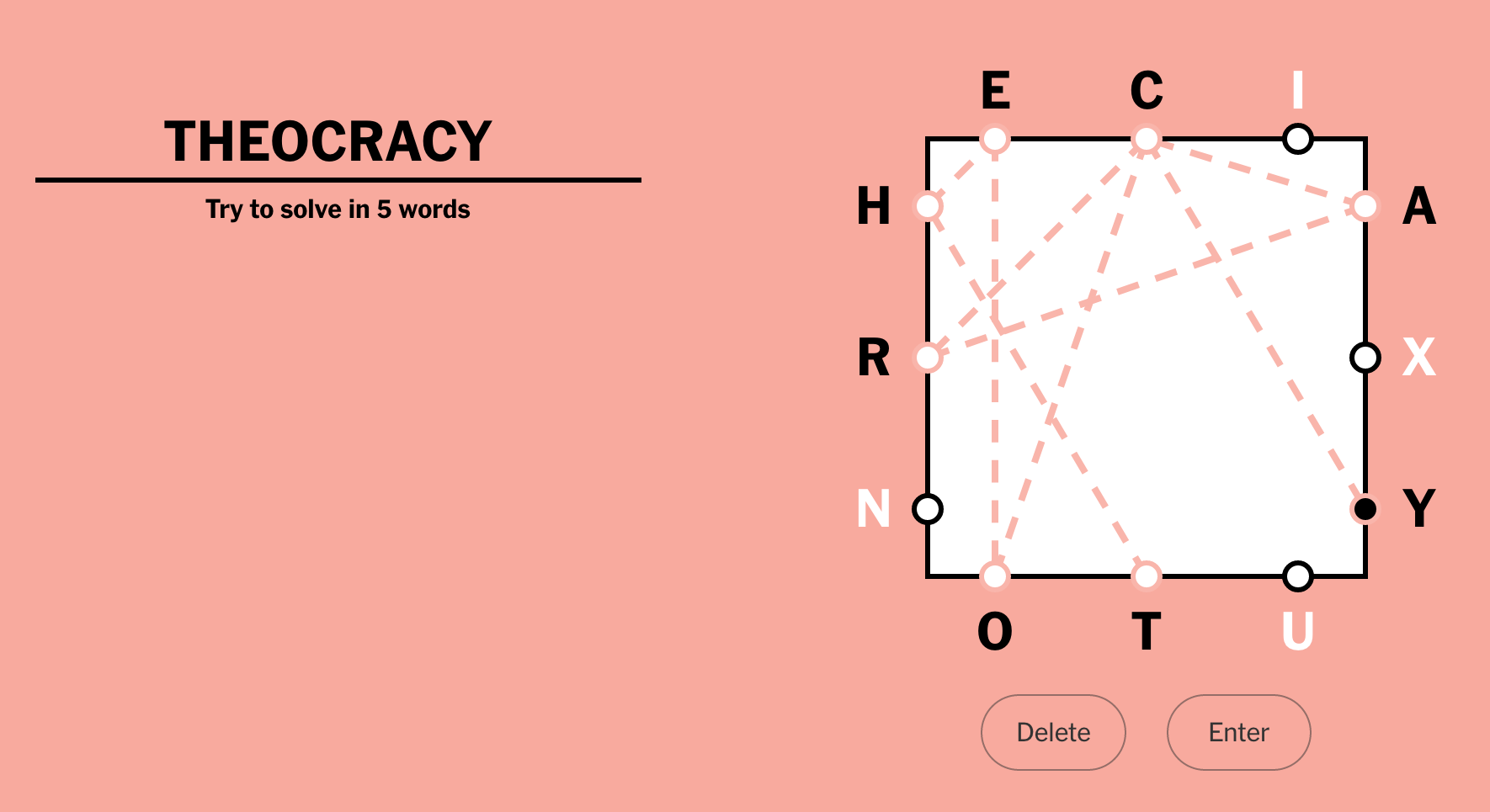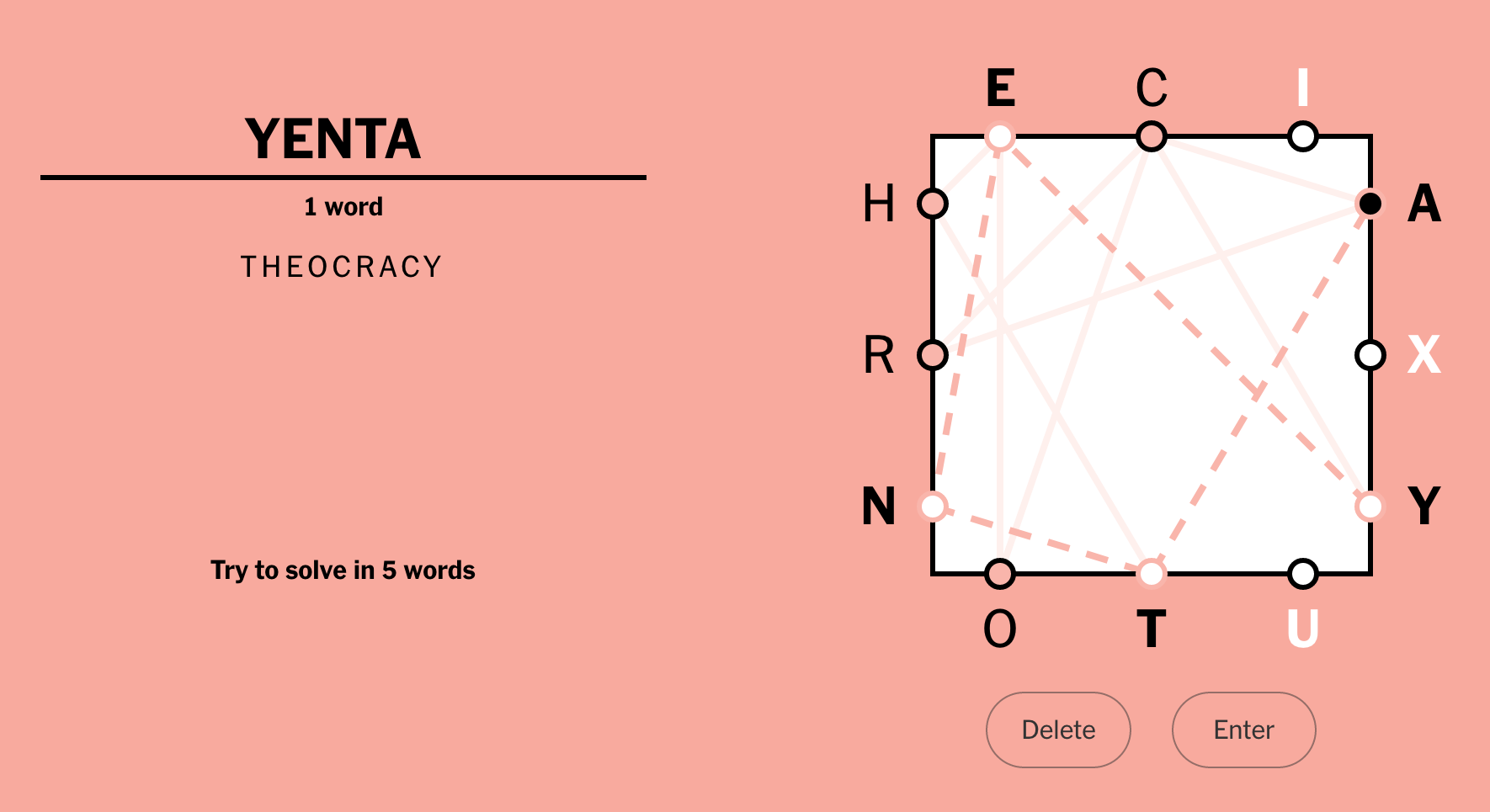# Letter boxed

With more time on my hands during this quarantine time, I started doing the Letter Boxed puzzle. You are given a square with three letters on each side:You form words by choosing letters in sequence. The only rule is that the next letter must be on a different side of the square than the previous letter:The goal is to use all the letters in a certain number of words. For this puzzle, the challenge is five words.

But if you look at the answers they provide for yesterday’s puzzle, it’s always just two words. So now I’m tormenting myself trying to find two-word solutions. And of course I started thinking about writing a program to find them.

I found a giant list of words and started hacking on some code. I wasn’t sure if I’d need some fancy tree structure for searching the solution space. I figured I would start simpler than that, and maybe it would work.

My strategy was to whittle down the list of words a few steps at a time. First, keep only the words formed from just the 12 letters in the puzzle. Then reduce the list to only those that can be formed following the “not same side” rule. Then find pairs of words that end and start with the same letter, and use all 12 letters:

`import collectionsimport itertoolsimport sysdef print_sample(label, words, n=10):    print(f"{label}: {len(words)} words: {', '.join(itertools.islice(words, n))}")with open("words2.txt") as fwords:    words = set(w.strip() for w in fwords)print_sample("All words", words)sides = sys.argv[1:]alphabet = set("".join(sides))words = {w for w in words if set(w) < alphabet}print_sample("Only the letters", words)numbered_sides = {c: i for i, side in enumerate(sides) for c in side}def is_possible(word):    for first, second in zip(word, word[1:]):        if numbered_sides[first] == numbered_sides[second]:            return False    return Truepossible = {w for w in words if is_possible(w)}print_sample("Possible words", possible)starts = collections.defaultdict(list)for word in possible:    starts[word].append(word)print("Solutions:")for word1 in possible:    last = word1[-1]    for word2 in starts[last]:        if set(word1 + word2) == alphabet:            print(word1, word2)`

This code is just stream-of-consciousness coding, intermixing running statements with function I needed. On some previous puzzles, it came up with way too many solutions. For “riu pgh lcs yao” it found 1002 pairs of words! Solutions like “hypacusia argol” are not satisfying...

My giant list of words has 466,551 words. I also have a smaller file with only 45,404 words. I refactored the code to make it more flexible. Now it will try the small word list first, and only go to the second larger list if there are no solutions:

`import collectionsimport itertoolsimport sysdef print_sample(label, words, n=10):    print(f"{label}: {len(words)} words: {', '.join(itertools.islice(words, n))}")class LetterBoxed:    def __init__(self, sides):        self.sides = sides        self.alphabet = set("".join(sides))        self.numbered_sides = {c: i for i, side in enumerate(self.sides) for c in side}    def is_possible(self, word):        for first, second in zip(word, word[1:]):            if self.numbered_sides[first] == self.numbered_sides[second]:                return False        return True    def solutions(self, words):        alpha_words = {w for w in words if set(w) < self.alphabet}        print_sample("Using only the letters", alpha_words)        possible = {w for w in alpha_words if self.is_possible(w)}        print_sample("Possible words", possible)        starts = collections.defaultdict(list)        for word in possible:            starts[word].append(word)        for word1 in possible:            last = word1[-1]            for word2 in starts[last]:                if set(word1 + word2) == self.alphabet:                    yield (word1, word2)def main(sides):    letter_boxed = LetterBoxed(sides)    for wordfile in ["words.txt", "words2.txt"]:        with open(wordfile) as fwords:            words = set(w.strip() for w in fwords)        print_sample("All words", words)        solutions = list(letter_boxed.solutions(words))        if not solutions:            print("No solutions with these words")            continue        print(f"{len(solutions)} solutions:")        for word1, word2 in solutions:            print(word1, word2)        breakif __name__ == "__main__":    main(sys.argv[1:])`

With this, “riu pgh lcs yao” finds five solutions from the short word list, using words I actually know, like “gracious splashy.”

Unfortunately, sometimes the official solution uses words that aren’t in even the enormous word list. A previous puzzle was “tub pxi snq oja”, and the solution offered was “juxtaposition niqab,” which is frustrating. Playing word puzzles inevitably brings you face to face with the differences between accepted word lists.

After I wrote my code, I found:

• Caleb Robinson’s blog post about his solution, which involved the fancier tree structures I avoided.
• Art Steinmetz’s blog post about both generating the puzzle and solving it, in R. He mentions the generalizations of different numbers of sides, and numbers of letters per side. I was amused to realize that my code doesn’t care how many sides or letters per side are used, so it works for any number.

Just for grins, I’m wondering if there’s some crazy way to abuse regexes to do most of the work. Too much time on my hands...

»  8 reactionsWhere in your code does it account dor not using lettees from the same sides?@Mikhail: the is_possible function uses numbered_sides to check that adjacent letters are not from the same side of the square.For a regex-based solution, I think you have to make one thing the search space and the other thing the pattern. You can go either way: puzzle→pattern, words→space, or words→pattern, puzzle→space.

For puzzle→pattern, the tree of possibilities generalizes to 12! = 479001600 (it's less because of grouping into edges, but the full factorial is certainly an upper bound). That number is far too large for a single regex of nested (?:a|b) style alternatives.

Making a pattern from the word list would face similar challenges. At least you would only have to do it once, not for each puzzle!

I think what this means, ultimately, is that you can't really make a single regex that will search either space to find a solution in one pass. That makes this exercise somewhat less satisfying...

You can still use regexes to filter the words and possible solutions before running a non-regex checker to speed things up.

For example, using the 12-letter alphabet from your example puzzle:
```^[acehinortuxy]{1,12}\$
```
Applying that to my input word list reduces the count from 370104 to 8765.

You can also filter the words that have the right numbers of letters. Here is a check that ensures a word has at most one letter "a"
`^(?:[^a]*+a){0,1}[^a]*+\$`
(That uses possessive quantifiers to avoid catastrophic backtracking, but they aren't available in the built-in Python re... You can get possessive quantifiers in the external Python regex module in PyPI.)

If that regex seems a little overcooked, for example {0,1} is the same as ?, it's because you'd want to build the regex programmatically from the puzzle input, and it should work even if there are two or three a's available. Here is a longer version that considers all the available letters. This uses forward-lookaheads because the letters can be in any order:
```(?x) ^
(?= (?:[^a]*+a){0,1} [^a]*+ \$)
(?= (?:[^c]*+c){0,1} [^c]*+ \$)
(?= (?:[^e]*+e){0,1} [^e]*+ \$)
(?= (?:[^h]*+h){0,1} [^h]*+ \$)
(?= (?:[^i]*+i){0,1} [^i]*+ \$)
(?= (?:[^n]*+n){0,1} [^n]*+ \$)
(?= (?:[^o]*+o){0,1} [^o]*+ \$)
(?= (?:[^r]*+r){0,1} [^r]*+ \$)
(?= (?:[^t]*+t){0,1} [^t]*+ \$)
(?= (?:[^u]*+u){0,1} [^u]*+ \$)
(?= (?:[^x]*+x){0,1} [^x]*+ \$)
```
Applying that reduces the potential words contributing to a solution from 8765 to 2193.

One more filter you can apply is making sure that candidate words follow the edge-to-edge rules, meaning that you don't use two letters in a row from one edge of the puzzle. For example, considering the ECI edge of your sample puzzle, it must be followed by one of the letters from the other edges:
```[cei][ahnortuxy]
```
To make this check an entire word, we need to consider all the source and destination edges, plus use forward lookaheads to avoid consuming more than one character at a time:
```(?x) ^
(?: (?= [cei](?:\$|[ahnortuxy]) |
[axy](?:\$|[cehinortu]) |
[otu](?:\$|[acehinrxy]) |
[hnr](?:\$|[aceiotuxy])
)
. # consume the char
)*
\$
```
Applying that pattern reduces the potential words from 2193 to 1213. It doesn't check whether letters have already been used, but when you combine it with the other checks, the list is narrowed considerably before you need to resort to a non-regex algorithm for the final step.Your code assumes that no letter appears twice. Is that a safe assumption?@Aron: it's going to take me quite some time to digest that! (If I *should* digest it...)

@Julian: you are right, my code won't handle duplicated letters. The puzzle online seem to never have them, so I'm OK with the limitation.Well, you know that "wondering if there’s some crazy way to abuse regexes" is my siren song. What did you expect? ?

The tl;dr though: I don't see a way to solve with regexes, but you can use them to reduce the solution space before running a non-regex algorithm.here's my pass at a regex solution. turned out not to be as terrible as i'd imagined. the trick is to filter OUT bad sequences. eliminating words that have chars outside the set of good chars first and then eliminating anything with a repeated char from any one side keeps the regex sane.

inputs are a list of sides and the path to a word list (like '/usr/share/dict/words').
```def legal_words(sides, word_list):
# "Illegal" regexes. If any match then the word is not usable.
#   `tooshort` is less than 3 characters.
#   `badletters` is any letter not on the puzzle board.
#   `badsequence` means a repeated letter on the same side of the board.
tooshort = r'^.{1,2}\$'
badletters = '[^' + ''.join(set(''.join(sides))) + ']'
badsequences = '|'.join(['[' + side + '](?=[' + side + '])' for side in sides])
return [word for word in Path(word_list).read_text().split()
if not regex.search(word)]
```
so, for instance today's letter list is:
```sides = ['utp', 'aef', 'mli', 'bor']
```
which creates the regex:
```re.compile(r'^.{1,2}\$|[^temapubrfilo]|[utp](?=[utp])|[aef](?=[aef])|[mli](?=[mli])|[bor](?=[bor])',
re.IGNORECASE|re.UNICODE)
```This is all cheating!